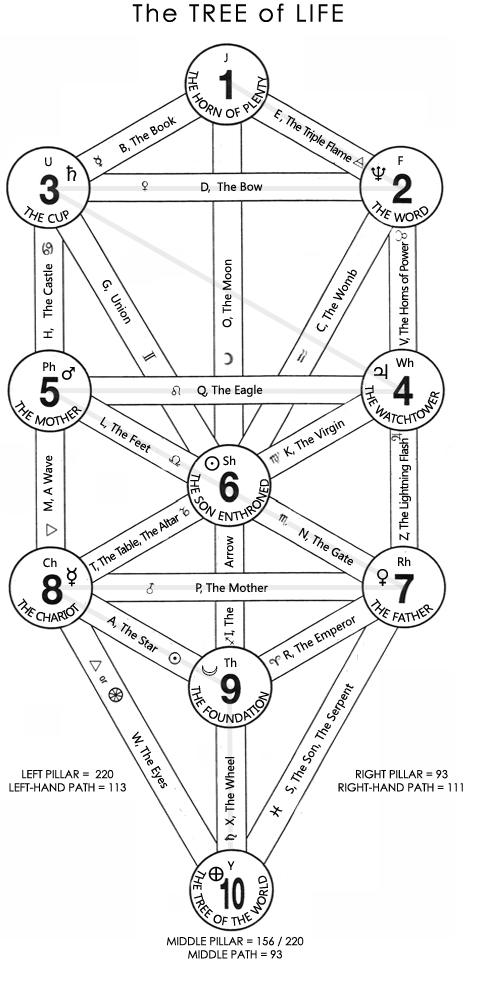# The Tree of Life# Notes

C's hard, O's full, F's active.

## Demonstration

(1) The Sum of the Sephiras, that is the Letters of all three Pillars when summed, are equal to the gematria for “Tree of Life” which is equivalent to the Middle Path:

(Left Pillar = 77) + (Right Pillar = 93) + (Middle Pillar = 204) = 384 = ‘Tree of Life’ = 109 + 210 + 65 = OIX (middle Path) = 80 + 4 + 300

(2) Gematria for 'Middle Pillar' is equivalent to the sum of the Sephiras in the Middle Pillar:

Middle Pillar” = (40 + 4 + 8 + 8 + 50 + 1) + (3 + 4 + 50 + 50 + 4 + 9) = 231 = H + Sh + Th + Y (the middle pillar)

(3) Both Left- and Right-hand paths are equivalent to 111, and when summed are equivalent to a phase of the Middle Pillar.

Left Path = B + J + M + W = 6 + 5 + 40 + 60 =111

Right Path = E + V + Z + S = 1 + 80 + 6 + 24 = 111

Middle Pillar = H + Sh + Th + Y = 7 + 49 + 96 + 70 = 222

(4) The Letters of the middle path combined with the letters of either the right-hand or left-hand path and summed are equal to a phase of the middle pillar:

H + Sh + Th + Y = 7 + 31 + 96 + 70 = 204 = 93 + 111, i.e. the middle path plus either the left or right pillar.

Etc.

## Observations

PATHS

• Left Path : B + J + M + W = 6 + 5 + 40 + 60 =111
• Right Path : E + V + Z + S = 1 + 80 + 6 + 24 = 111
• Middle Path : O + I + X = 93 / 213 / 384

PILLARS

Left Pillar : C + Ch + Ph = 30 + 37 + 10 = 77

Right Pillar : F +Rh + Wh = 10 + 16 + 67 = 93

Middle Pillar:

• T final: H + Sh + Th + Y = 7 + 31 + 96 + 70 = 204 (= 93 + 111, i.e. the middle path plus either the left or right pillar)
• S final: H + Sh + Th + Y = 7 + 49 + 105 + 70 = 231 = “Middle Pillar” = (Note: In this case, the sum of all sephiras are 231 + 77 + 93 = 401 when F is active and C is hard, and S final)
• S & T final: H + Sh + Th + Y = 7 + 49 + 96 + 70 = 222 = 111 + 111, i.e. the sum of both left and right paths.